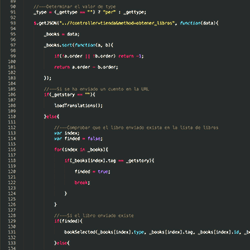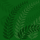# str_replace PHP function in JavaScript

Working with JavaScript, if one wants to replace a part of a string with another string, usually, the replace String method is used. This method allows to manipulate a string using another string or a regular expression. Next it is shown how it is used:

### Using a string

```var str = "I have a cat and a dog but my cat doesn't like my dog";
console.log( str.replace("cat", "kitten") );
// I have a kitten and a dog but my cat doesn't like my dog
```

### Using a regular expression

```var str = "I have a cat and a dog but my cat doesn't like my dog";
console.log( str.replace(/cat/g, "kitten") );
// I have a kitten and a dog but my kitten doesn't like my dog
```

If one needs to replace more than one value, normally multiple concatenated replace methods are used using regular expressions to achieve this:

### Replace multiple times

```var str = "I have a cat and a dog but my cat doesn't like my dog";
console.log( str.replace(/have/, "had").replace(/doesn't/, "didn't").replace(/cat/g, "kitten") );
// I had a kitten and a dog but my kitten didn't like my dog
```

This method has a deficiency. Using this cumulative replacement it is possible to replace an already replaced string, for example:

```var str = "I have a cat and a dog but my cat doesn't like my dog";
console.log( str.replace(/cat/g, "dog").replace(/dog/g, "cat") );
// I have a cat and a cat but my cat doesn't like my cat
```

The previous snippet should return I have a dog and a cat but my dog doesn’t like my cat, but cat was replaced with dog at the beginning, and the second replace changed all the dogs for cats.

There is a function in PHP that allows changing sub-strings using strings or arrays as parameters. If arrays are used, all the occurrences in the first array are replaced by the ones in the second and the replacement is not cumulative. This function is str_replace. So, this function is more versatile than the JavaScript multiple replace methods solution. So, let’s replicate the str_replace function in JavaScript:

### Replicating the PHP str_replace function in JavaScript

```function str_replace(\$search, \$replace, \$subject) {

// Create the regular expression to find the occurrences
var reg;

// If the \$search parameter is a string
if ( typeof(\$search) == "string" ) {

// Escape all the characters used by regular expressions
\$search = \$search.replace(/[.?*+^\$[\]\\(){}|-]/g, "\\");
reg = new RegExp("(" + \$search + ")", "g");

} else {

// Escape all the characters used by regular expressions
\$search = \$search.map(function(i) {
return i.replace(/[.?*+^\$[\]\\(){}|-]/g, "\\");
});

reg = new RegExp("(" + \$search.join("|") + ")", "g");

}

// Create the replacement
var rep;

// If the \$search parameter is a string
if ( typeof(\$replace) == "string" ) {
rep = \$replace;
} else {
// If the \$search is a string and the \$replace parameter an array
if ( typeof(\$search) == "string" ) {
rep = \$replace;
} else {
// If the \$search and \$replace parameter are arrays
rep = function (i) {
return \$replace[ \$search.indexOf(i) ];
}
}
}

return \$subject.replace(reg, rep);

}
```

### Compressed JavaScript str_replace function

```function str_replace(\$f, \$r, \$s){
return \$s.replace(new RegExp("(" + (typeof(\$f) == "string" ? \$f.replace(/[.?*+^\$[\]\\(){}|-]/g, "\\") : \$f.map(function(i){return i.replace(/[.?*+^\$[\]\\(){}|-]/g, "\\")}).join("|")) + ")", "g"), typeof(\$r) == "string" ? \$r : typeof(\$f) == "string" ? \$r : function(i){ return \$r[\$f.indexOf(i)]});
}
```

We can use this function in the next way:

```var str = "I have a cat and a dog but my cat doesn't like my dog";
console.log( str_replace(["cat", "dog"], ["dog", "cat"], str) );
// I have a dog and a cat but my dog doesn't like my cat
```
I wrote this function some time ago, inspired in a question on StackOverflow. There are also other valuable solutions there.(1 votes, average: 5.00 Out Of 5)str_replace PHP function in JavaScriptarray,javascript,regular-expressions,snippet,string
1.akmal says:
•elchininet says: